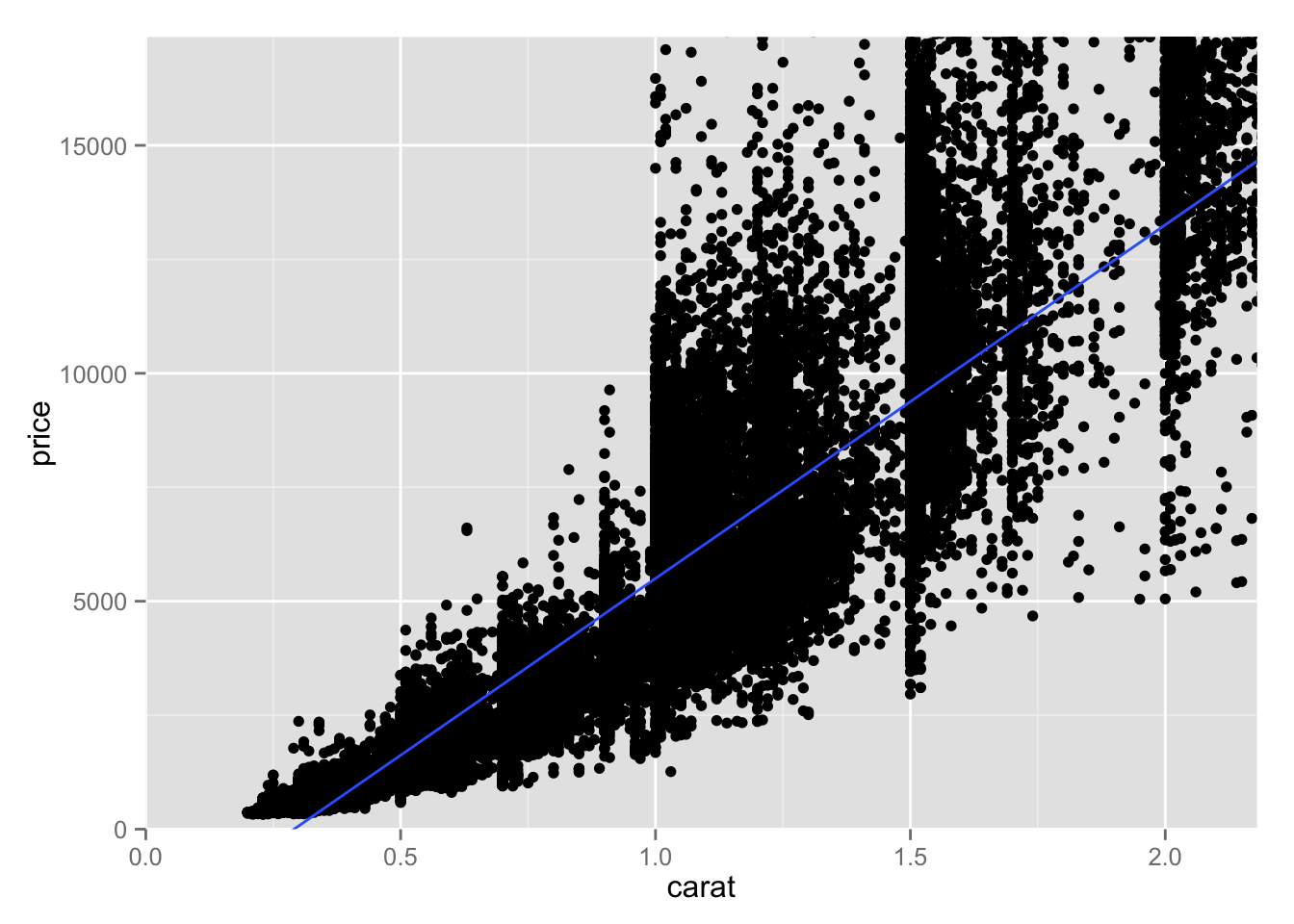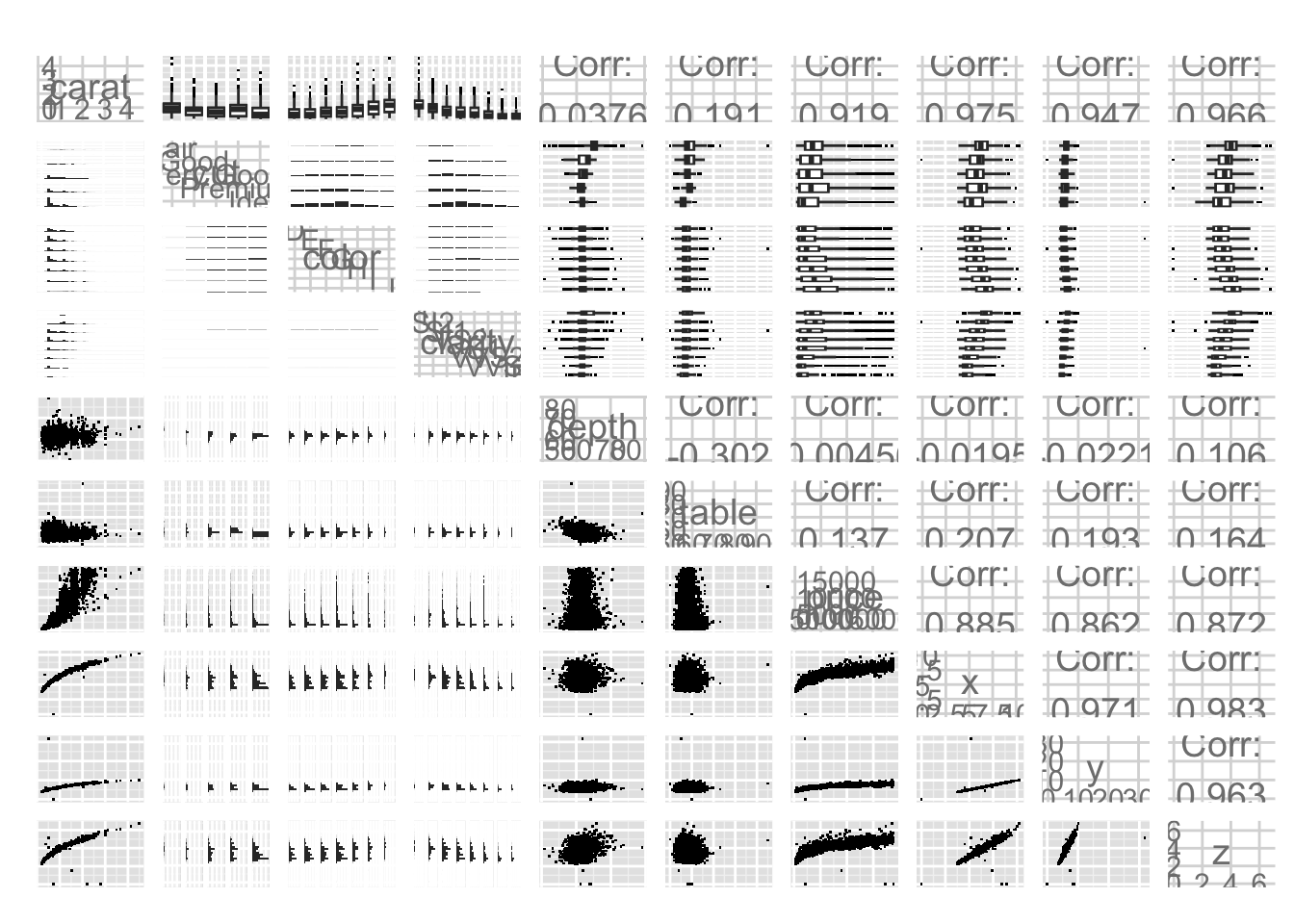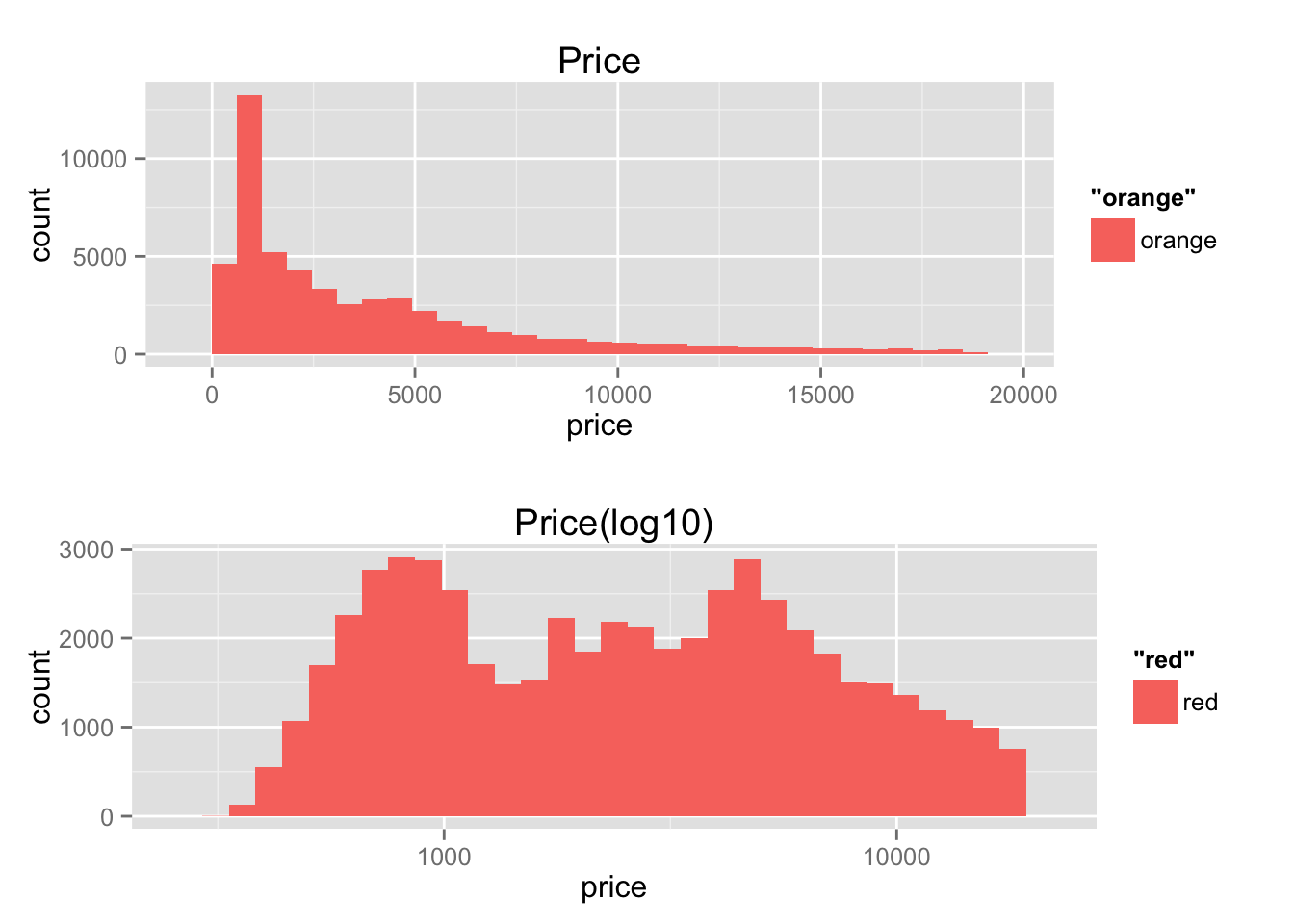# Diamonds Analysis

|   Source

We will explore diamonds dataset, history, and use EDA to create quantitative analysis.

### Welcome

• Salomon, data analyst at Facebook, will make EDA to explore diamond.
• In the end, we will know, given the diamonds, is it a good deal or not.
• Wel also be able to predict the price of given diamonds.

### Scatterplot Review

``````library(ggplot2)
data(diamonds)
names(diamonds)``````
``````##   "carat"   "cut"     "color"   "clarity" "depth"   "table"   "price"
##   "x"       "y"       "z"``````
``````ggplot(aes(x=carat, y = price),
data = diamonds)+
geom_point()+
coord_cartesian(xlim=c(0,quantile(diamonds\$carat,0.99)),
ylim=c(0,quantile(diamonds\$price,0.99)))+
stat_smooth(method = "lm")``````### Price and Carat Relationship

• There are fix relationship between carat and price
• Same carat may have higher price, but it depends on the other variables
• More weight of carat, the higher price, but not go any lower

• We can see that some exponential increase as the price go higher.
• diversion increase as carat higher and price higher.
• By using linear model, we may have off predicting the price(too bias!)

### Frances Gerety

• We can’t just input the diamond data and pop the price.
• The diamonds’ price itself has each background story related to it.
• First found south africa.
• Earlier diamonds only found in India and Brazil. Back then, diamonds only priced by its supply.
• Then the biggest diamonds cartel build in US and control the diamonds market, De Beers which advertise the diamonds in many other way

#### A diamonds is….. FOREVER

• Diamonds earlier only for the rich, but the slogan, which made by Frances Gerety, quote “A diamonds is forever” which point to enggagement should make diamond engagement ring.

### The Rise of Diamonds

• The slogan itself is powerful. It create the intense of the diamonds.
• They do that, as earlier said, the company has create a cartel and monopolize the diamonds in South Africa.
• Since then they give movie star a diamond, price vary giving each other between selebrity.
• They can even make Britsh Royal to use diamonds in their crown over other gems.
• They create the engagement ring should wear diamonds. And advertise what are the price of diamonds compared to what men achieve in life.
• Movie engagement most contain diamond

### ggpairs Function

• each variable plotting other variable in ggpairs
• qual qual, scat qual auan
• group histogram in top left qual-qual group by x
• boxplot qual-quan
• correlation at lower right quan-quan
``````# install these if necessary
# install.packages('GGally')
# install.packages('scales')
# install.packages('memisc')
# install.packages('lattice')
# install.packages('MASS')
# install.packages('car')
# install.packages('reshape')
# install.packages('plyr')

# load the ggplot graphics package and the others
library(ggplot2)
library(GGally)
library(scales)
library(memisc)``````
``````## Loading required package: lattice
##
## Attaching package: 'memisc'
##
## The following object is masked from 'package:scales':
##
##     percent
##
## The following objects are masked from 'package:stats':
##
##     contr.sum, contr.treatment, contrasts
##
## The following object is masked from 'package:base':
##
##     as.array``````
``````# sample 10,000 diamonds from the data set
set.seed(20022012)
diamond_samp <- diamonds[sample(1:length(diamonds\$price), 10000), ]
ggpairs(diamond_samp, params = c(shape = I('.'), outlier.shape = I('.')))``````What are some things you notice in the ggpairs output?

• price and carat is highly correlated shown by close to 1 at cor.test function.
• Synthesizing varibles(merging) may make useful analsysis

### The Demand of Diamonds

``library(gridExtra)``
``## Loading required package: grid``
``````plot1 <- ggplot(aes(x=price),
data = diamonds,
)+
geom_histogram(aes(fill='orange'))+
ggtitle('Price')
#scale_fill_brewer(aes(color='qual'))

plot2 <- ggplot(aes(x=price),
data = diamonds)+
geom_histogram(aes(fill='red'))+
scale_x_log10()+
ggtitle('Price(log10)')
# scale_fill_brewer(aes(color='qual'))

grid.arrange(plot1,plot2)``````
``````## stat_bin: binwidth defaulted to range/30. Use 'binwidth = x' to adjust this.
## stat_bin: binwidth defaulted to range/30. Use 'binwidth = x' to adjust this.``````### Connecting Demand and Price Distributions

• Notice that by transforming into log 10 we can see our usual normal distribution.
• Followed by two peak in the middle that is binomial distribution.
• Notice that mid-split in the plot(also shown later in the middle). This will also shows how divided the the people with less money and more money

### Scatterplot Transformation

• By using cuberoot function that we made, we are able to transform our exponential model into linear model.

### Create a new function to transform the carat variable

``````library(scales)
cuberoot_trans = function() trans_new('cuberoot',
transform = function(x) x^(1/3),
inverse = function(x) x^3)``````

#### Use the cuberoot_trans function

``````# ggplot(aes(carat, price), data = diamonds) +
#   geom_point() +
#   scale_x_continuous(trans = cuberoot_trans(), limits = c(0.2, 3),
#                      breaks = c(0.2, 0.5, 1, 2, 3)) +
#   scale_y_continuous(trans = log10_trans(), limits = c(350, 15000),
#                      breaks = c(350, 1000, 5000, 10000, 15000)) +
#   ggtitle('Price (log10) by Cube-Root of Carat')``````

### Overplotting Revisited

• As we learn earlier, overplotting means obscure our keypoints that maybe there somewhere in the plot
• If take a look at our data, we can see the top of our data by using sorting and head over the highest data.
``head(sort(table(diamonds\$price), decreasing=T))``
``````##
## 605 802 625 828 776 698
## 132 127 126 125 124 121``````
``head(sort(table(diamonds\$carat), decreasing=T))``
``````##
##  0.3 0.31 1.01  0.7 0.32    1
## 2604 2249 2242 1981 1840 1558``````
• Overplotting can simply encounter with jitter or alpha

Add a layer to adjust the features of the scatterplot. Set the transparency to one half, the size to three-fourths, and jitter the points.

``````ggplot(aes(carat, price), data = diamonds) +
geom_point(position='jitter',size=0.75,alpha=1/2) +
scale_x_continuous(trans = cuberoot_trans(), limits = c(0.2, 3),
breaks = c(0.2, 0.5, 1, 2, 3)) +
scale_y_continuous(trans = log10_trans(), limits = c(350, 15000),
breaks = c(350, 1000, 5000, 10000, 15000)) +
ggtitle('Price (log10) by Cube-Root of Carat')``````
``## Warning: Removed 1691 rows containing missing values (geom_point).``